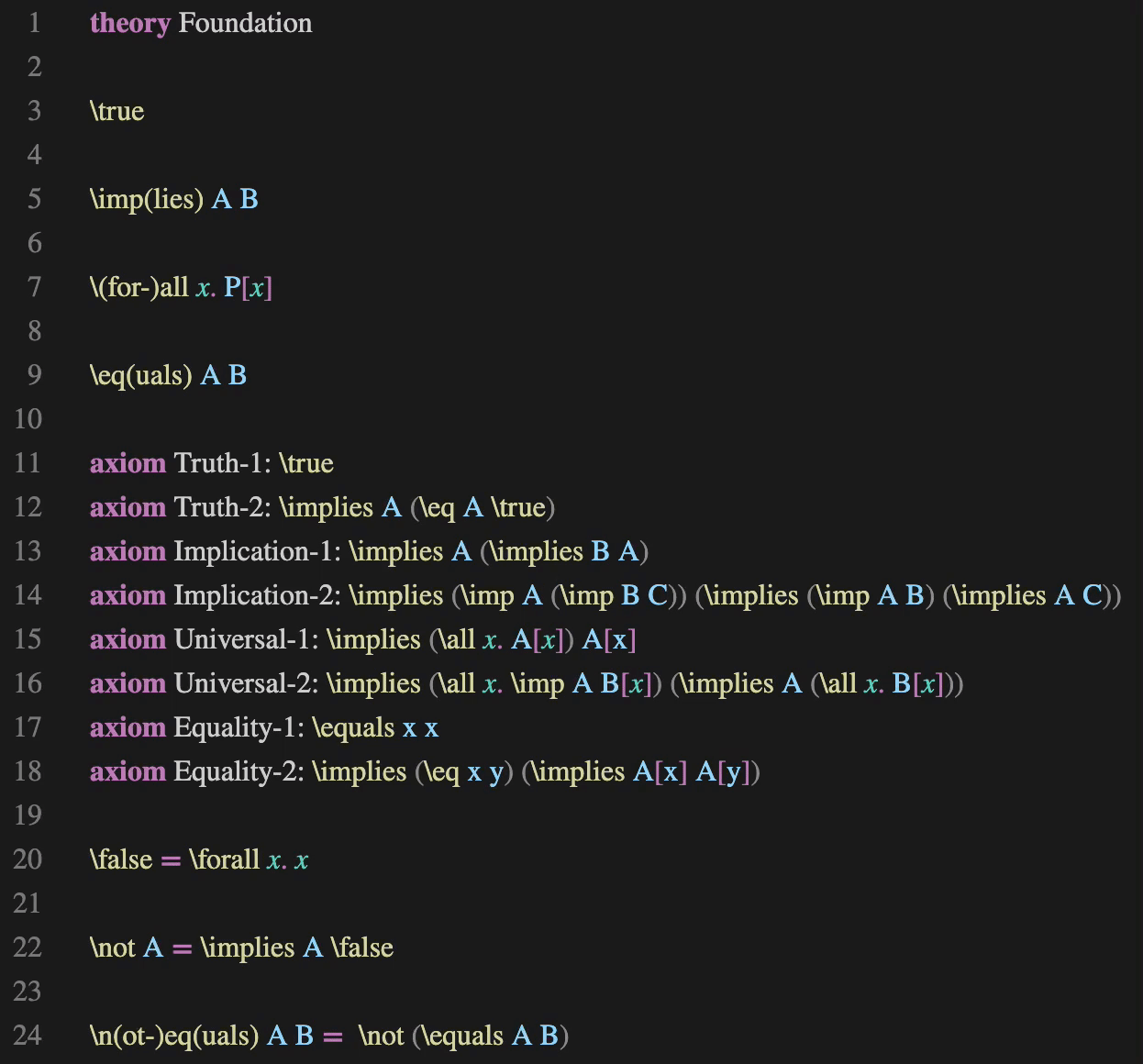# Axioms Are Inference Rules

by Steven Obua

February 5, 2023

In my last post I have described in detail the theory Foundation.practal, which I planned to become the basis of any theory development in Practal. A version without custom syntax is displayed again below:The idea was that any other theory had to directly or indirectly import this foundational theory. As such, Foundation.practal would be special. Therefore I didn’t consider it a problem that Practal would need to treat the abstractions \implies and \for-all in a special way which is not apparent from looking at Foundation.practal alone: out of Abstraction Logic’s four proof rules, two have been specially designed to help capture the meaning of \implies and \for-all:

• Modus ponens: Given theorems \implies A B and A, the theorem B can be deduced.
• Introduction of universal quantification: Given the theorem A[x], the theorem \for-all x. A[x] can be deduced. Note that this inference rule cannot be simply represented as the axiom \implies A[x] (\for-all x. A[x]), as this axiom actually describes the existential quantifier, not the universal one.

In the semantics of Abstraction Logic, these special rules are represented by the move from general abstraction algebras to logic algebras.

The other two proof rules, axiom invocation and substitution, are generic and do not depend in any way on the particular abstractions involved.

This morning I started to think about how to organize theory import and modules. At some point I realized that my current concept of abstraction logic is too narrow. Instead of defining an abstraction logic as a logic signature together with a list of axioms, I should rather define it as follows:

An abstraction logic is a signature together with a list of inference rules.

This definition eliminates any mention of special abstractions like \implies and \for-all, and replaces the list of axioms by a list of inference rules. Axioms are just inference rules without any premisses, so this redefinition of our notion of abstraction logic strictly generalizes the old one.

Of course, the success of this new definition depends on whether we can represent inference rules similarly straightforwardly as we represent axioms. An axiom is just a term. An inference rule consists of a conclusion and a list of premisses. The conclusion is just a term, while a premise is a template $$[x_1, \ldots, x_n.\ t]$$. The semantic idea is that the $$x_1, \ldots, x_n$$ are universally quantified over.

The inference rules for modus ponens and universal quantification could then be written like this:

• Modus ponens

rule Modus-ponens:
premise \implies A B
premise A
infer B
• Introduction of universal quantification

rule Universal-quantification-intro:
premise x. A[x]
infer \for-all x. A[x]

We can thus replace the previous four proof rules by just two proof rules, one rule for substitution, and the other one for applying inference rules. It is even possible to go one step further, and to replace the proof rule for substitution by an inference rule:

• Substitution

rule Substitution:
premise x. A[x]
infer A[x]

This leaves us with a single proof rule, the one for applying inference rules!

It should be relatively easy to implement inference rules for Practal. Of course, we still need to carefully check if the theory of Abstraction Logic holds up under these changes. Ideally, we do that by formalizing Abstraction Logic in Practal!

While Foundation.practal is of course still a special theory in that it forms a complete logical system (at least if all the details turn out to be correct), and internally, Practal might optimize it, from an external point of view Foundation.practal is now a theory just like any other. This is good news for theory import and for modularization!

Even under this new definition, Abstraction Logic has still a proper model-theoretic semantics. So these inference rules are really axioms after all; it is just that our first take on how the axioms of Abstraction Logic look like was too simple.

If you’ve read so far, and like the direction Practal is going, please consider sponsoring Practal. I am now working full-time on Practal, and would like to continue to do so! I am also happy to give talks about Practal and Abstraction Logic either remotely or on-site. If you’d like to leverage Practal for your business, I am available for consulting services. I speak both English and German, and am happy to communicate in either of these languages.

You can contact me either through Practal’s sponsoring page, or directly via hi@practal.com.